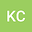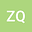Numerical approximation of basic reproduction number for an age-structured HIV infection model with both virus-to-cell and cell-to-cell transmissions
••• Kangkang Chang,
• zhang qimin
Kangkang Chang
Ningxia University

Corresponding Author:changkangkang86@sina.com

Author Profilezhang qimin
School Mathematics and Computer Science, Ningxia University,
Author Profile## Abstract

In general, the basic reproduction number (R0) cannot be explicitly calculated for HIV(Human Immunodeficiency Virus) infection model with age-structured in a infinite dimensional spaces. To find R0, we need to transform the HIV model into a finite-dimensional space. In this paper, we are absorbed in numerical approximation of R0, which is the non-negative dominant eigenvalues of the positive irreducible matrices whose spectrum radius is defined as the next generation matrix. The linear operators generated by infected population are discretized into ordinary differential equations in a finite n-dimensional space. Thus, the abstract problem is transformed to find the positive dominant eigenvalues of the next generation matrix, we obtain a threshold R_0,n. Based on the spectral approximation theory, we show that R_0,n →R0 as n →+∞. Finally, by virtue of a numerical simulation, we demonstrate the results of the theorem.
08 Oct 2020Submitted to Mathematical Methods in the Applied Sciences
09 Oct 2020Submission Checks Completed
09 Oct 2020Assigned to Editor
20 Oct 2020Reviewer(s) Assigned
02 Feb 2021Review(s) Completed, Editorial Evaluation Pending
03 Feb 2021Editorial Decision: Revise Major